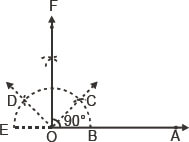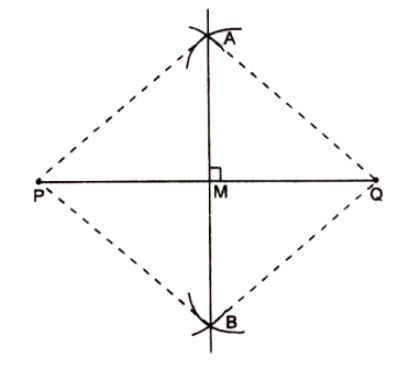# AP Board Class 9 Maths Chapter 13 Geometrical Constructions

Andhra Pradesh board Class 9 Maths Chapter 13, deals with the topic of constructions. In this chapter, students will learn about the steps of drawing the perfect geometrical figures and also writing the justification for it. They will also learn how to extract the needed values and complete the required shape. Some of the topics that are covered in the AP Board Class 9 Maths 13th Chapter Construction are:

• Basic Constructions.
• Constructions of a triangle.
• Construction of Circle Segment

In this AP Board 13th Chapter Class 10 Maths students will learn to construct geometrical figures, such as a line segment, an angle, a triangle, a quadrilateral and also some basic constructions justified with proofs.

## What is Construction?

A construction basically involves drawing shapes, angles and lines in perfection. Geometrical constructions are usually carried out using an ungraduated ruler, compass and a pencil.

### Basic Constructions

The main objective of this chapter is to give the process of necessary logical proofs to all basic constructions. Some of the constructions include;

• The perpendicular bisector of a given line segment.
• The bisector of a given angle.
• Construction of 60° angle at the initial point of a given ray.

## Construction of Triangles

While the topic is already taught in earlier standards, in class 9 students learn how to construct triangles when special types of measures are given.

• Construct a triangle when its base, a base angle and the sum of other two sides are given.
• Construct a triangle when its base, a base angle and the difference of the other two sides are given.
• Construct a triangle, when its perimeter and its two base angles are given.

Students can check out some of the chapter questions along with their solutions to know more about the concept.

Question 1: Construct an angle of 90° at the initial point of a given ray.Solution:

• Draw a ray OA.
• Taking 0 as the centre and suitable radius a semicircle has to be drawn cutting OA at B.
• With the same radius, the semicircle should be divided into three equal parts such that
$$\begin{array}{l}\widetilde{BC}=\widetilde{CD}=\widetilde{DE}\end{array}$$
• Draw
$$\begin{array}{l}\overrightarrow{OC} and \overrightarrow{OD}\end{array}$$
• Draw
$$\begin{array}{l}\overrightarrow{OF}, the bisector of \angle COD\end{array}$$

Thus, ∠AOF = 90o.

Question 2: Construct the perpendicular bisector of a given line segment.

Steps of Construction:

• Draw arcs with P as the centre and any line as the suitable radius on each side of PQ.
• Similarly, with q as the centre and taking same radius draw arcs on each side of PQ that will cut the arcs at A and B.
• Join AB to meet PQ at point M so that AB bisects PQ at the point M and becomes perpendicular to PQ. However, AB is the required perpendicular bisector.Know more about Andhra Pradesh Board and access various study materials including syllabus, exam timetable, etc. at BYJU’s.